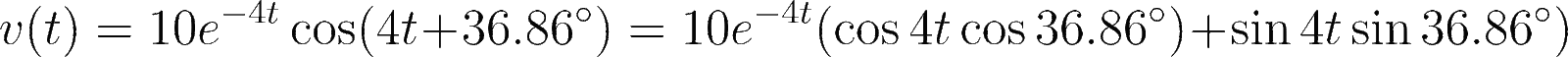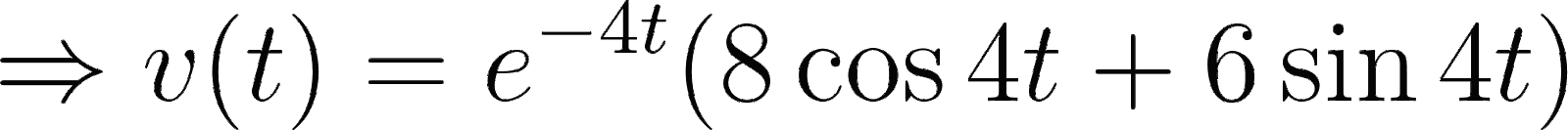Introduction To Electric Circuits - 9 Edition - Chapter 14.12 - Problem P14.2-3
Register Now

Join StudySoup

Get Full Access to Introduction To Electric Circuits - 9 Edition - Chapter 14.12 - Problem P14.2-39781118477502

# Figure P 14.12-3 shows a circuit represented in (a) the time domain and (b) the

Introduction to Electric Circuits | 9th Edition

Problem P14.2-3

Figure P 14.12-3 shows a circuit represented in (a) the time domain and (b) the frequency domain, using Laplace transforms. An incorrect analysis of this circuit indicates that IL s s 2 s2 s 5 and VC s 20 s 2 s s 2 s 5 (a) Use the initial and final value theorems to identify the error in the analysis. (b) Correct the error. Hint: Apparently, the error occurred as VC(s) was calculated from IL(s).

Accepted Solution
Step-by-Step Solution:

Step 1 of 3

Given,###### Chapter 14.12, Problem P14.2-3 is Solved

Step 2 of 3

Step 3 of 3

Unlock Textbook Solution